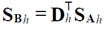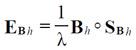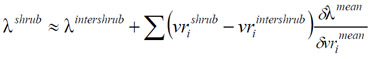### Alden B. Griffith. 2010. Positive effects of native shrubs on Bromus tectorum demography. Ecology 91:141–154.

Appendix B. Methods for sensitivity, elasticity, and life table response experiment (LTRE) analyses.

Following Caswell (2001), I calculated the sensitivity matrix for each period as,(B.1)

where DhT is the transpose of the product of the periodic matrices used to calculate Ah, excluding Bh. For example, for h = 1, Dh = B3 B2. SAh is the sensitivity matrix of Ah equaling vwT where v and w are vectors of the reproductive values and stable stage distribution associated with Ah, respectively (v and w are scaled so that their scalar product is equals 1). I then used the chain rule on matrix elements to calculate sensitivities of individual vital rates.& Elasticity matrices for each period are calculated as,(B.2)

where ◦ indicates element-by-element multiplication.

The LTRE analysis for each shrub microhabitat is,(B.3)

where vri is the ith vital rate and λmean is calculated using the mean shrub and intershrub vital rates (vrmean). In other words, λshrub is proportional to λinterhsrub plus the summed contributions of each vital rate difference. Essentially, the contribution of each vital rate to population growth is the product of its treatment-induced difference and mean sensitivity.

LITERATURE CITED

Caswell, H. 2001. Matrix population models: construction, analysis, and interpretation. Second edition. Sinauer Associates, Sunderland, Massachusetts, USA.

[Back to E091-013]